# 10.3 Discussion and Exercises

The implicit representation of a complete binary tree as an array, or list, seems to have been first proposed by Eytzinger , as a representation for pedigree family trees. The BinaryHeap data structure described here was first introduced by Williams .

The randomized MeldableHeap data structure described here appears to have first been proposed by Gambin and Malinowski . Other meldable heap implementations exist, including leftist heaps [16,48, Section 5.3.2], binomial heaps , Fibonacci heaps , pairing heaps , and skew heaps , although none of these are as simple as the MeldableHeap structure.

Some of the above structures also support a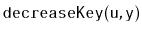operation in which the value stored at node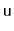is decreased to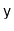. (It is a pre-condition that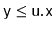.) This operation can be implemented intime in most of the above structures by removing nodeand adding. However, some of these structures can implementmore efficiently. In particular,takes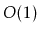amortized time in Fibonacci heaps andamortized time in a special version of pairing heaps . This more efficientoperation has applications in speeding up several graph algorithms including Dijkstra's shortest path algorithm .

Exercise 10..1   Illustrate the addition of the values 7 and then 3 to the BinaryHeap shown at the end of Figure 10.2.

Exercise 10..2   Illustrate the removal of the next two values (6 and 8) on the BinaryHeap shown at the end of Figure 10.3.

Exercise 10..3   Implement the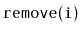method, that removes the value stored inin a BinaryHeap. This method should run intime. Next, explain why this method is not likely to be useful.

Exercise 10..4   A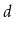-ary tree is a generalization of a binary tree in which each internal node haschildren. Using Eytzinger's method it is also possible to represent complete-ary trees using arrays. Work out the equations that, given an index, determine the index of's parent and each of'schildren in this representation.

Exercise 10..5   Using what you learned in Exercise 10.4, design and implement a DaryHeap, the-ary generalization of a BinaryHeap. Analyze the running times of operations on a DaryHeap and test the performance of your DaryHeap implementation against that of the BinaryHeap implementation given here.

Exercise 10..6   Illustrate the addition of the values 17 and then 82 in the MeldableHeap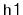shown in Figure 10.4. Use a coin to simulate a random bit when needed.

Exercise 10..7   Illustrate the removal of the next two values (4 and 8) in the MeldableHeapshown in Figure 10.4. Use a coin to simulate a random bit when needed.

Exercise 10..8   Implement the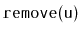method, that removes the nodefrom a MeldableHeap. This method should run inexpected time.

Exercise 10..9   Show how, in a BinaryHeap or MeldableHeap, to find the second smallest value in constant time.

Exercise 10..10   Show how, in a BinaryHeap or MeldableHeap, to find the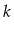th smallest value in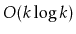time. (Hint: Using another heap might help.)

Exercise 10..11   Suppose you are given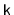sorted lists, of total length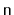. Show how, using a heap, to merge these into a single sorted list in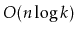time. (Hint: Starting with the casecan be instructive.)

opendatastructures.org